## Thursday, June 25, 2015

### Customizing your contMap style visualization using phytools: User questions & answers

A phytools user recently contacted me with the following questions about the phytools function `contMap` for mapping the evolution of continuous trait on the tree using a color gradient:

a) Does the “length” value in the legend specify how many millions of years the legend bar represents?
b) If so, is it possible to suppress the length call and and have an additional time scale across the whole tree instead?
c) Is there any way to name the trait value?
d) Is there a simple way to change the colour, on which one maps the trait?

Since these questions probably come up for other users as well, I thought I'd try to address them one by one here.

First, let's review the function `contMap`. Here is a quick demo illustrating it's use, which I will explain further below:

``````library(phytools)
## simulate a tree
tree<-pbtree(n=26,tip.label=LETTERS,scale=100)
plotTree(tree)
``````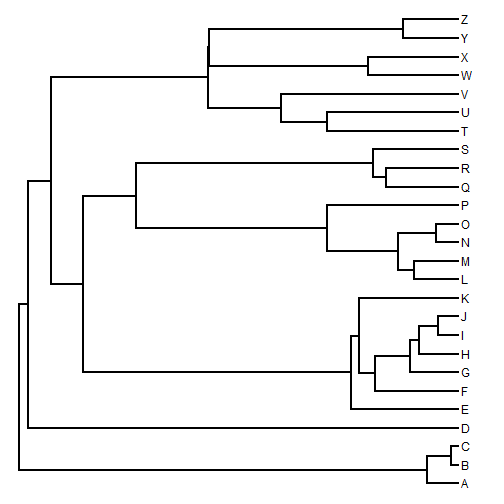``````## simulate some character data
x<-fastBM(tree,sig2=0.1)
x
``````
``````##          A          B          C          D          E          F
## -5.2978022 -6.1858831 -5.7305370 -2.0332898 -4.2643267 -0.8574406
##          G          H          I          J          K          L
## -2.2867929 -1.1968481 -0.8374673 -0.6996979 -3.6258800  2.2016812
##          M          N          O          P          Q          R
##  2.3865797  3.0411627  2.9741112  0.5108229  0.8685003  1.6050838
##          S          T          U          V          W          X
##  3.1910221 -0.5765872  1.0726064 -1.2610571 -0.5643860  0.4667727
##          Y          Z
##  2.5718508  2.5810971
``````
``````## generate an object of class "contMap"
obj<-contMap(tree,x,plot=FALSE)
obj
``````
``````## Object of class "contMap" containing:
##
## (1) A phylogenetic tree with 26 tips and 25 internal nodes.
##
## (2) A mapped continuous trait on the range (-6.185883, 3.191022).
``````
``````## plot it usin default settings:
plot(obj)
``````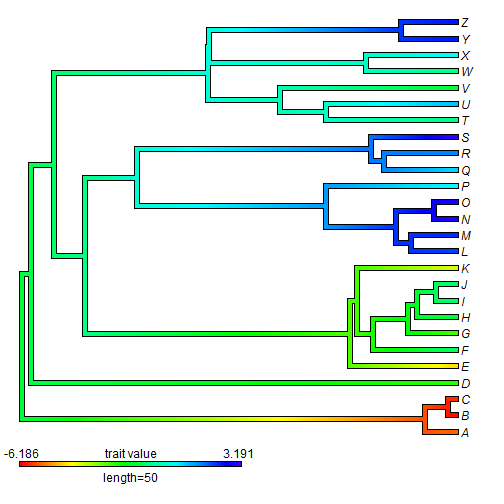The first question was: “Does the "length” value in the legend specify how many millions of years the legend bar represents?“

The answer is "yes” - if our branch lengths are in millions of years. Otherwise it will be in whatever units we have for the edge lengths of the tree. We can verify this by replotting our object of class `"contMap"`, while leaving enough space for a horizontal axis which we can then add to the plot for comparison. We can even change the length of the plotted legend as follows:

``````plot(obj,mar=c(5.1,0.2,0.2,0.2),legend=40)
axis(1)
title(xlab="time from the root")
``````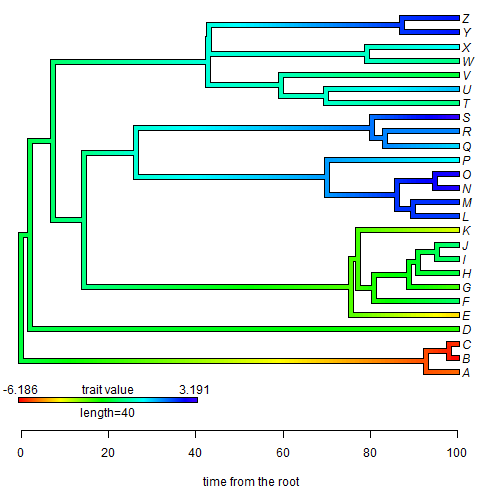The second question was then: “If so, is it possible to suppress the length call and and have an additional time scale across the whole tree instead?”

Well, I've just (above) demonstrated how to include a labeled x-axis in the plot. What I think the user really wants to do is suppress the text below the `contMap` legend. This is possible, but a little trickier. Here's how we can do it:

``````## first plot our tree sans legend
plot(obj,legend=FALSE,ylim=c(1-0.09*(Ntip(obj\$tree)-1),Ntip(obj\$tree)),
mar=c(5.1,0.4,0.4,0.4))
## now add our legend, but without the text underneath
lims=obj\$lims,digits=3,prompt=FALSE,x=0,
y=1-0.08*(Ntip(obj\$tree)-1),lwd=4,fsize=1,subtitle="")
axis(1)
title(xlab="time from the root")
``````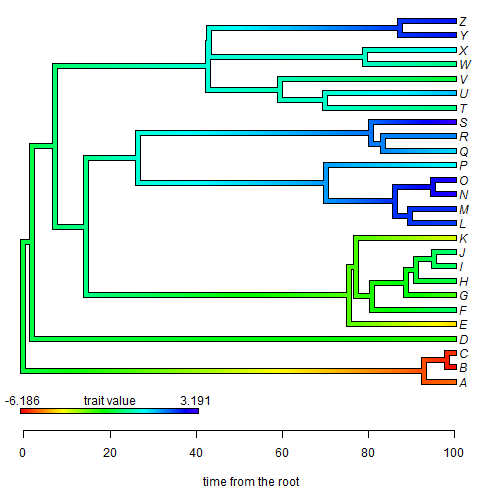The next question was: “Is there any way to name the trait value?”

Well - from the example above, it should be obvious that this is possible. Let's combine them together - but, let's call our trait (for example) log(body size):

``````plot(obj,legend=FALSE,ylim=c(1-0.09*(Ntip(obj\$tree)-1),Ntip(obj\$tree)),
mar=c(5.1,0.4,0.4,0.4))
lims=obj\$lims,digits=3,prompt=FALSE,x=0,
y=1-0.08*(Ntip(obj\$tree)-1),lwd=4,fsize=1,subtitle="")
axis(1)
title(xlab="time from the root")
``````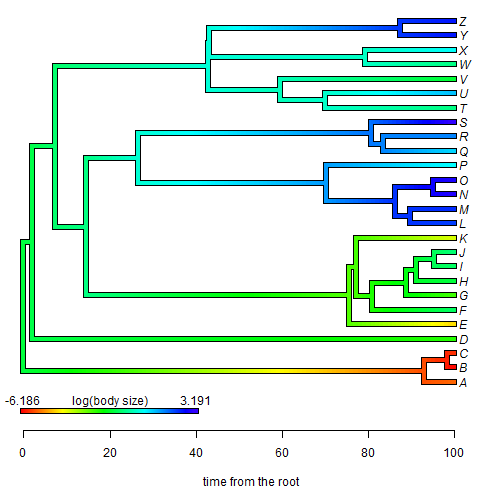Finally, the user asked: “Is there a simple way to change the colour, on which one maps the trait?” This one is actually pretty straightforward as there is a custom function in phytools, `setMap` for this. Let's change our rainbow color map to a blue-purple-red map:

``````obj<-setMap(obj,colors=c("blue","purple","red"))
## plot under default conditions
plot(obj)
``````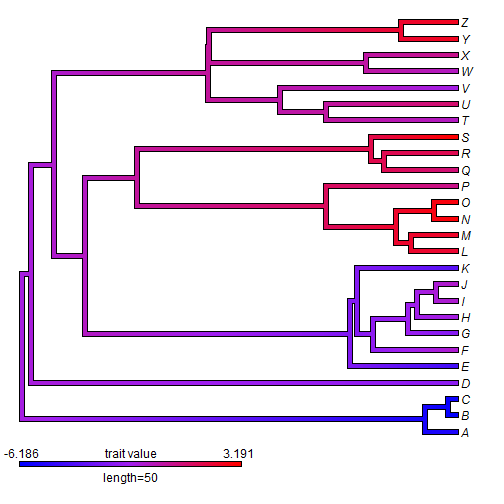1.I note that ape's axisPhylo() works on the contMap plot if users wanted a time-before-present time-scale but I noticed some odd rounding issues for the scale:

plot(obj,mar=c(5.1,0.2,0.2,0.2),legend=40)
axisPhylo()

Some odd interaction of phytools and ape code, maybe?

(Can send a jpg if you can't recreate it.)

2.This comment has been removed by the author.

3.it would be useful to have intermediate ticks in the trait value bar

1.Cool idea. Not sure when I'll get to posting this - but feel free to send me a reminder if you don't see it soon.

4.This comment has been removed by the author.

5.Is there a way to use serif fonts for the color bar and cladelabels?

1.6.